Atlanta Real Food is run by the Atlanta area chapter leaders of The Weston A. Price Foundation. Here you will find the latest news from local farmers, get information on how to properly prepare real foods, and stay up to date on local events.

Tuesday, February 5, 2019

Did you want to send your child to Farm Camp? \$10 off for our readers!!!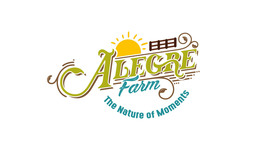In every walk with nature one receives far more than he seeks. - John Muir

 table div table+table+table+table div table{width:100%;padding:0}table div table+table+table+table div table img{width:96.23%;padding:0;float:none}table div table+table+table+table div table td{width:100%;padding:0 1.88% 18px}/* styles */A week of refreshing weather

 /* styles */ We hope that as the weather will be warm for the next days, you are able to enjoy the nature all around us. It is a therapy all on its own.table div table+table+table+table+table+table+table+table div table{width:100%;padding:0}table div table+table+table+table+table+table+table+table div table img{width:96.23%;padding:0;float:none}table div table+table+table+table+table+table+table+table div table td{width:100%;padding:0 1.88% 18px}/* styles */Our Weekly Volunteers

 /* styles */ On Mondays, we enjoy the company of our adult volunteers who come from Special Needs Schools of Gwinnett. They are truly able to embrace the beauty of nature and enjoy the animals while helping out with morning chores.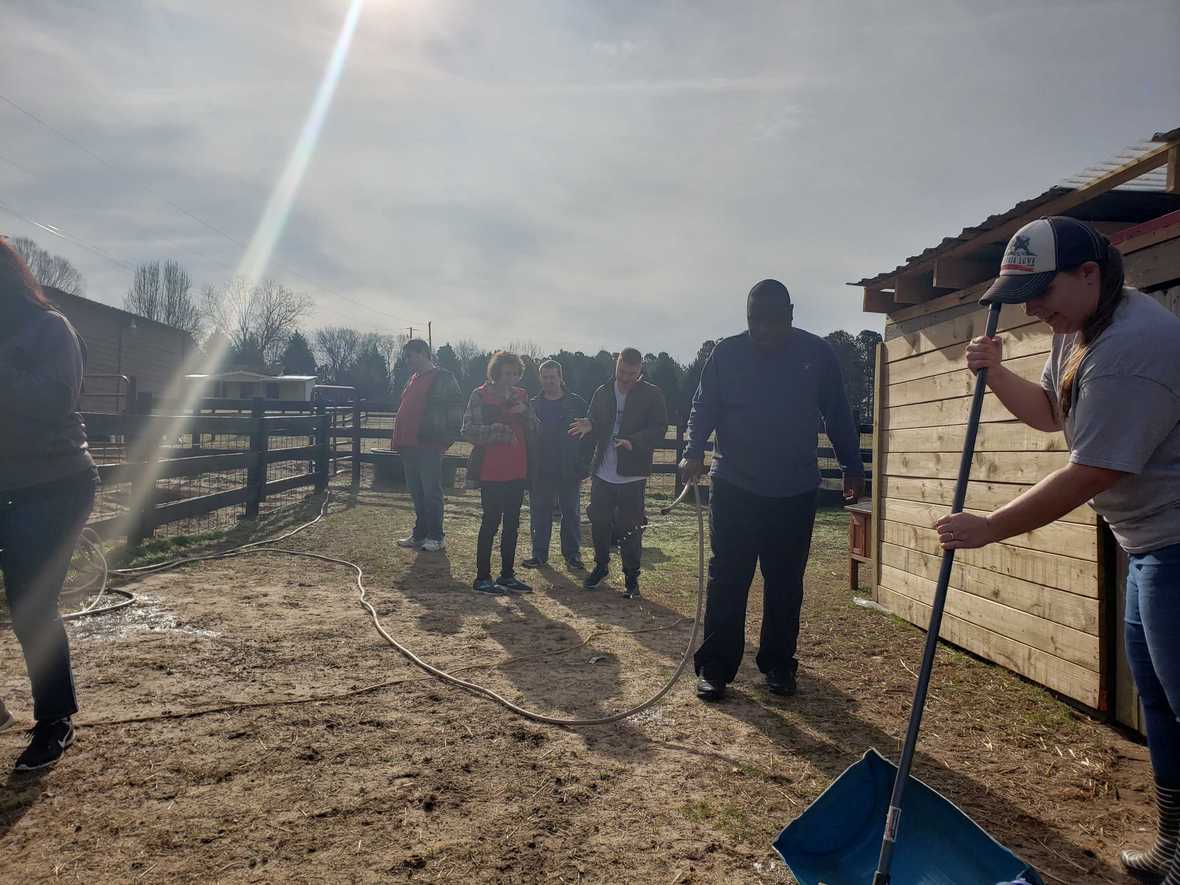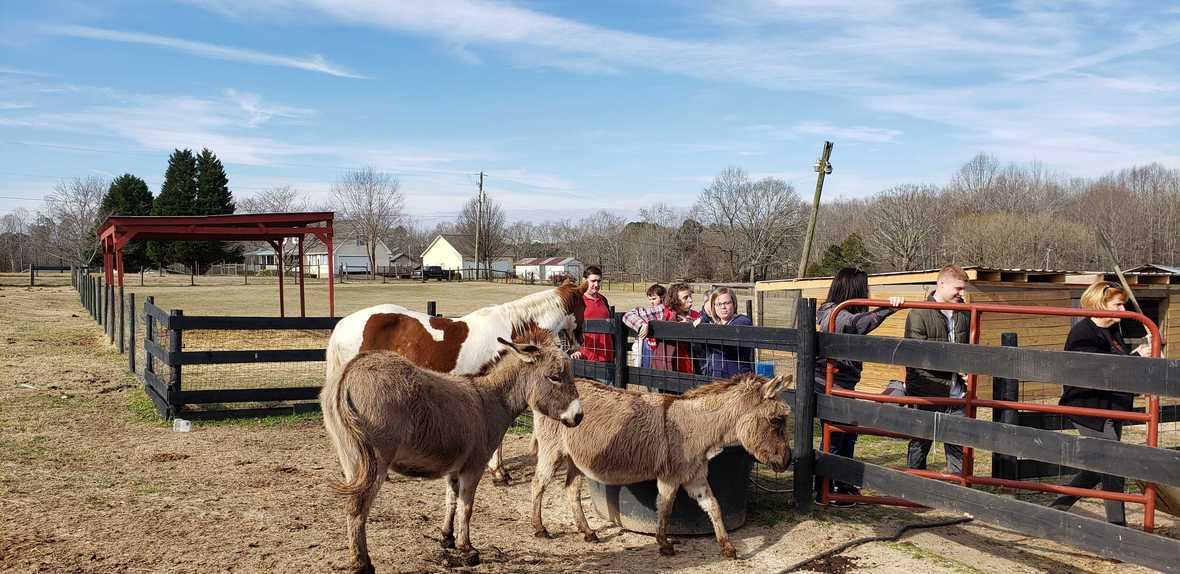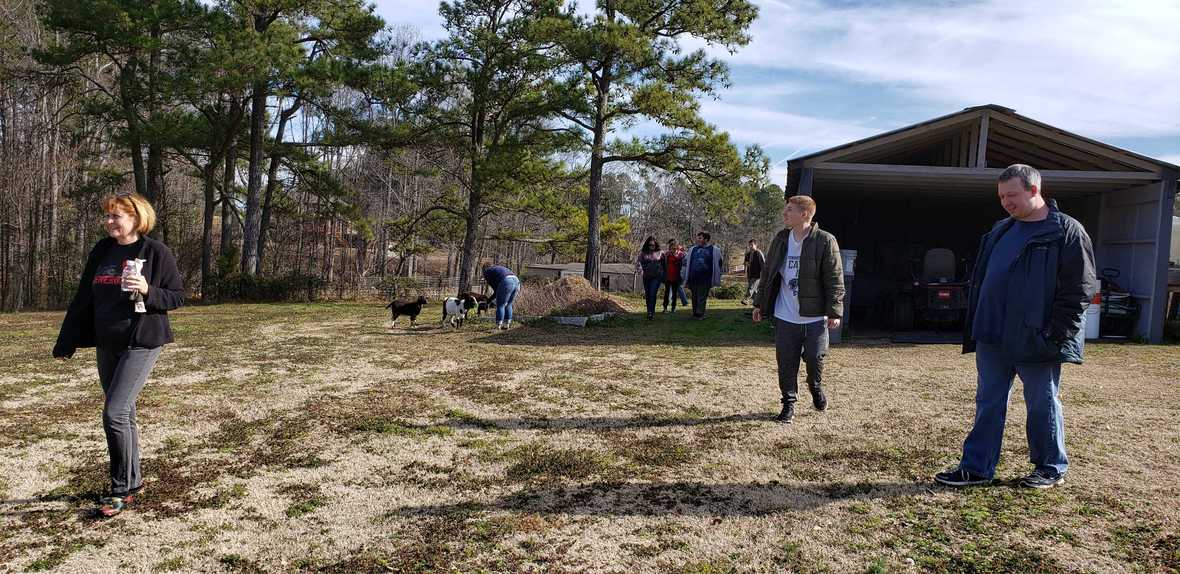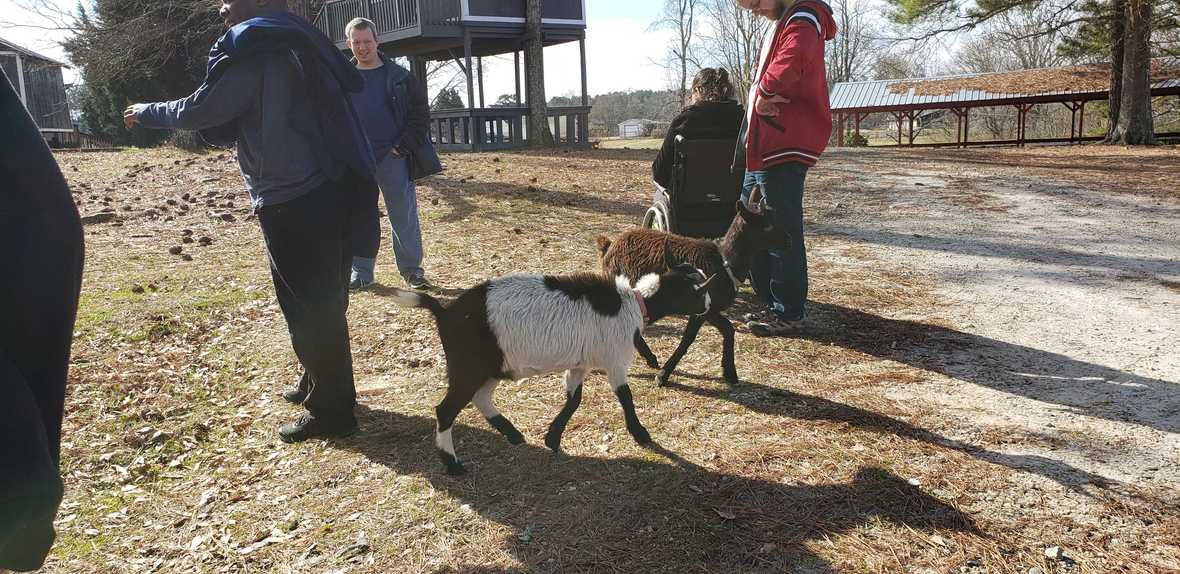/* styles */ Would you like your child to enjoy these activities. Let them join us at camp this Presidents' Day. Look at our upcoming events below for more information and how you can save \$10!!!
 table div table+table+table+table+table+table+table+table+table+table+table+table+table+table div table{width:100%;padding:0}table div table+table+table+table+table+table+table+table+table+table+table+table+table+table div table img{width:96.23%;padding:0;float:none}table div table+table+table+table+table+table+table+table+table+table+table+table+table+table div table td{width:100%;padding:0 1.88% 18px}/* styles */table div table+table+table+table+table+table+table+table+table+table+table+table+table+table+table+table div table{width:100%;padding:0}table div table+table+table+table+table+table+table+table+table+table+table+table+table+table+table+table div table img{width:96.23%;padding:0;float:none}table div table+table+table+table+table+table+table+table+table+table+table+table+table+table+table+table div table td{width:100%;padding:0 1.88% 18px}/* styles */\$10 off Presidents' Day Farm Camp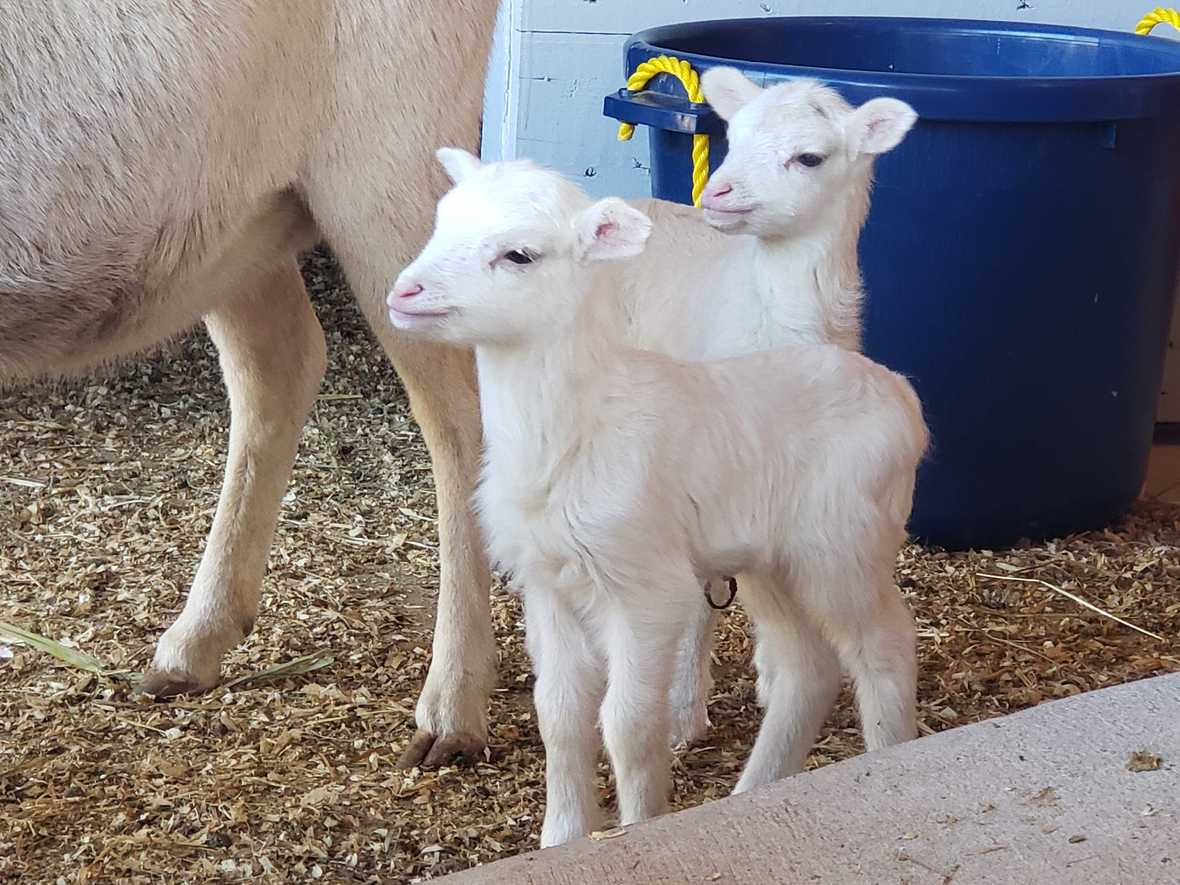Come meet our new lambs!

We can't believe we are doing this, but we're offering you, our loyal readers, \$10 off our Presidents' Day Farm Camp!

We have a few spots left, so don't delay in purchasing your tickets. To receive the discount, use this code when ordering: ThankYou19

This is a great experience for children to get outdoors and away from the electronics. It will give them a new perspective of nature through hands-on learning and absolute fun!

Let them interact with the farm animals while enjoying many fun activities!

Campers will:

Meet all the new babies being born!

Take a Hayride

Groom a Pony

Feed and Pet the Animals

 table div table+table+table+table+table+table+table+table+table+table+table+table+table+table+table+table+table+table+table+table div table{width:100%;padding:0}table div table+table+table+table+table+table+table+table+table+table+table+table+table+table+table+table+table+table+table+table div table img{width:96.23%;padding:0;float:none}table div table+table+table+table+table+table+table+table+table+table+table+table+table+table+table+table+table+table+table+table div table td{width:100%;padding:0 1.88% 18px}/* styles */Farm Adventure Day during Spring Break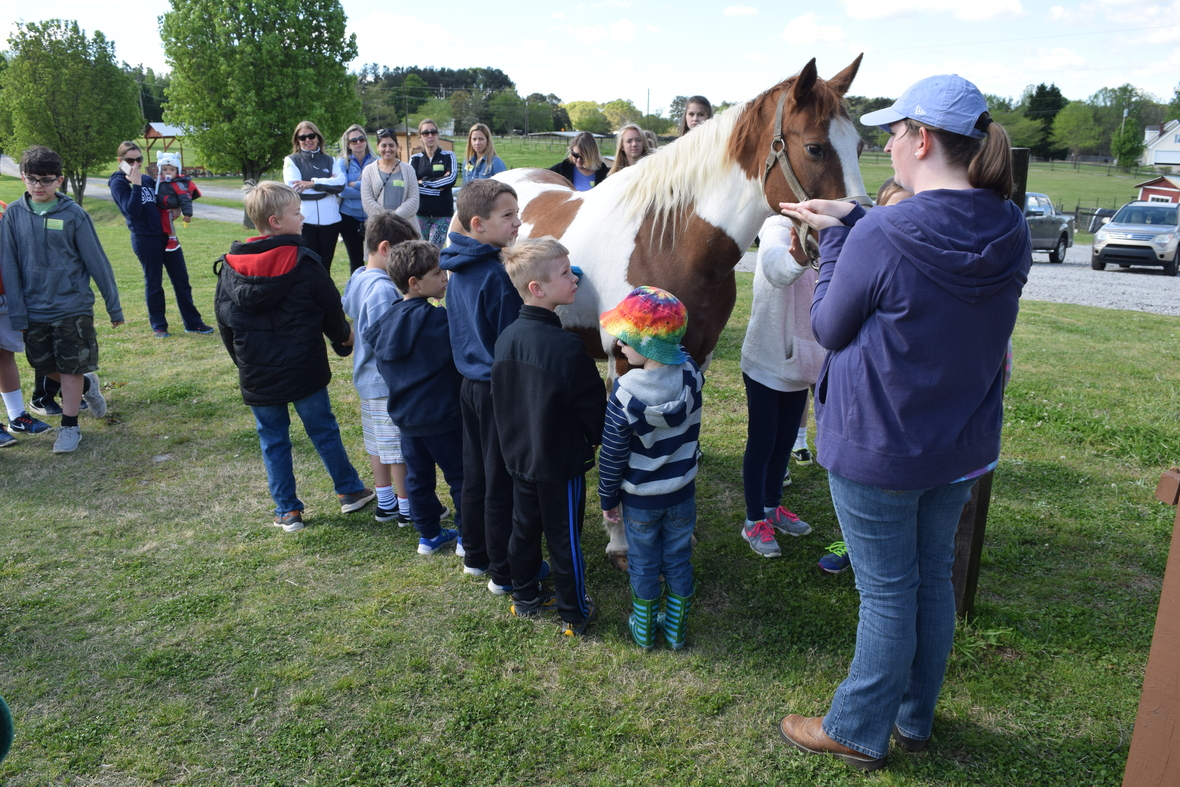Bring the whole family to Alegre Farm for fun and excitement this Spring Break!

10am-12pm on Friday, April 5th

Take a guided tour where you will meet new animals, smell the fresh flowers and herbs, learn how to make compost while the children have fun digging for worms, learn about our busy bees, go on a tractor ride!
Don't forget to bring your lunch to enjoy a picnic after the tour while your child can enjoy a pony ride!

Get Early Bird Pricing! Limited Tickets --- We will sell out quickly.

 table div table+table+table+table+table+table+table+table+table+table+table+table+table+table+table+table+table+table+table+table+table+table+table+table+table div table{width:100%;padding:0}table div table+table+table+table+table+table+table+table+table+table+table+table+table+table+table+table+table+table+table+table+table+table+table+table+table div table img{width:96.23%;padding:0;float:none}table div table+table+table+table+table+table+table+table+table+table+table+table+table+table+table+table+table+table+table+table+table+table+table+table+table div table td{width:100%;padding:0 1.88% 18px}/* styles */table div table+table+table+table+table+table+table+table+table+table+table+table+table+table+table+table+table+table+table+table+table+table+table+table+table+table div table{width:100%;padding:0}table div table+table+table+table+table+table+table+table+table+table+table+table+table+table+table+table+table+table+table+table+table+table+table+table+table+table div table img{width:96.23%;padding:0;float:none}table div table+table+table+table+table+table+table+table+table+table+table+table+table+table+table+table+table+table+table+table+table+table+table+table+table+table div table td{width:100%;padding:0 1.88% 18px}/* styles */Have a Dream Wedding to Plan?table div table+table+table+table+table+table+table+table+table+table+table+table+table+table+table+table+table+table+table+table+table+table+table+table+table+table+table+table+table div table{width:100%;padding:0}table div table+table+table+table+table+table+table+table+table+table+table+table+table+table+table+table+table+table+table+table+table+table+table+table+table+table+table+table+table div table img{width:96.23%;padding:0;float:none}table div table+table+table+table+table+table+table+table+table+table+table+table+table+table+table+table+table+table+table+table+table+table+table+table+table+table+table+table+table div table td{width:100%;padding:0 1.88% 18px}/* styles */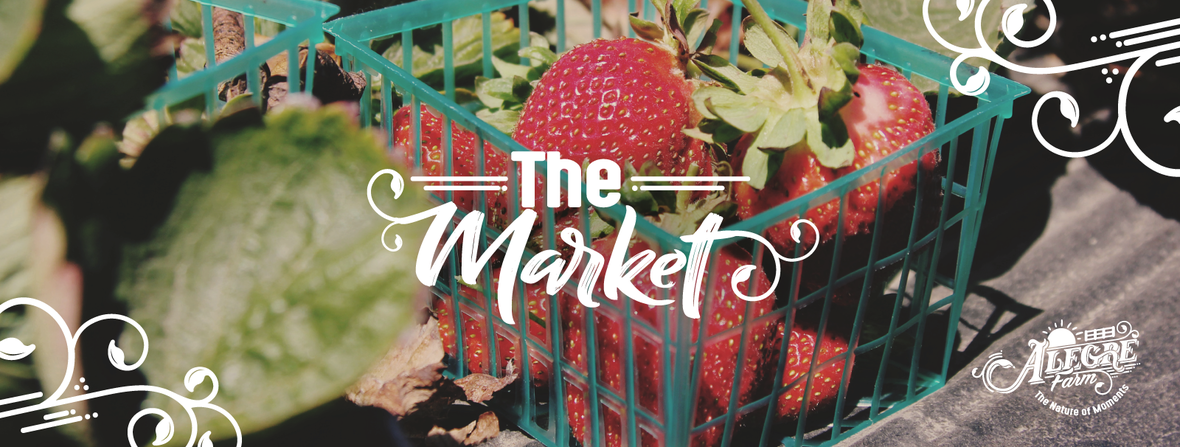Farmers Market... Fresh, Local, Better

 /* styles */ Support your local economy, Support your local farmer Please consider ordering from our online farmers market.raw milkfresh eggs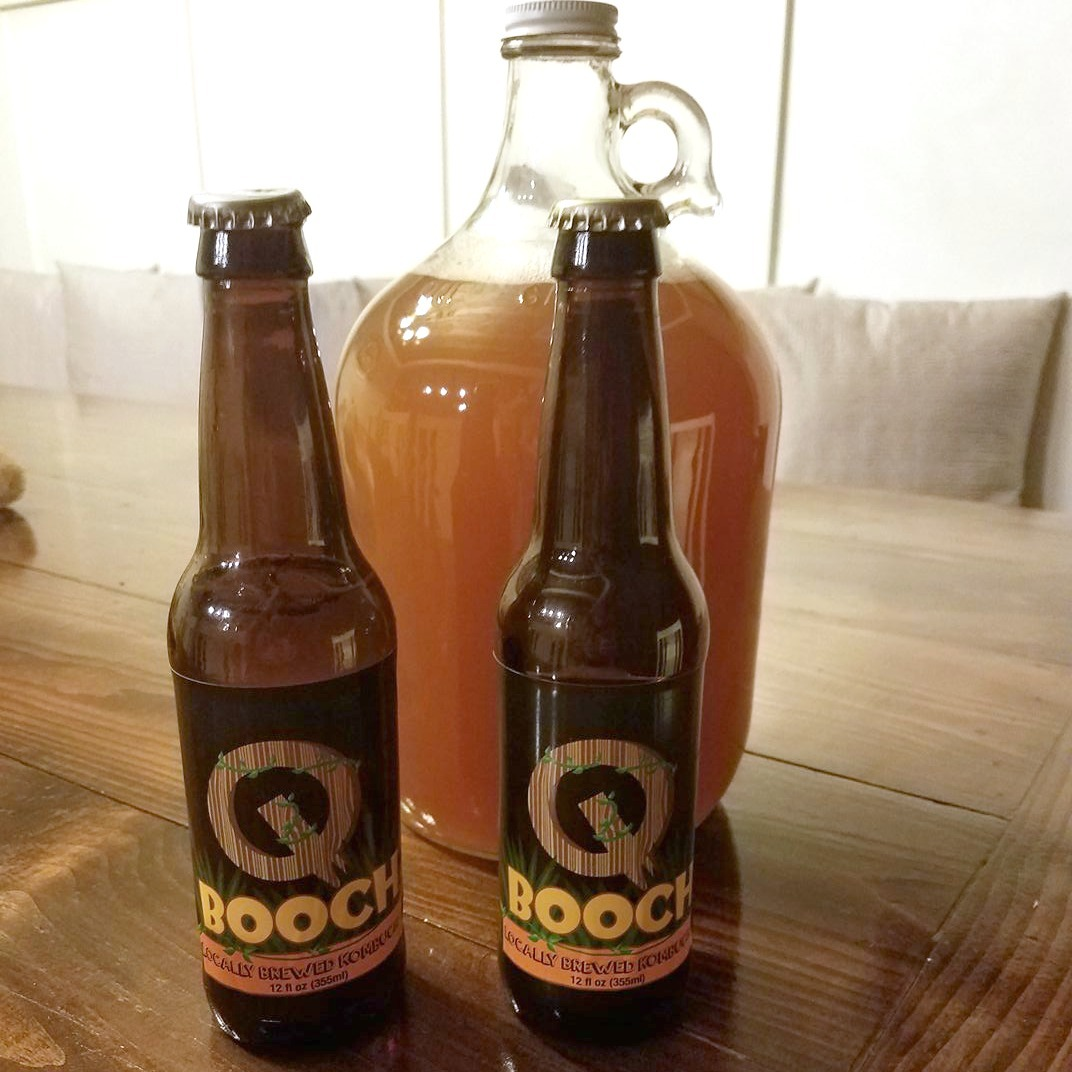kombucha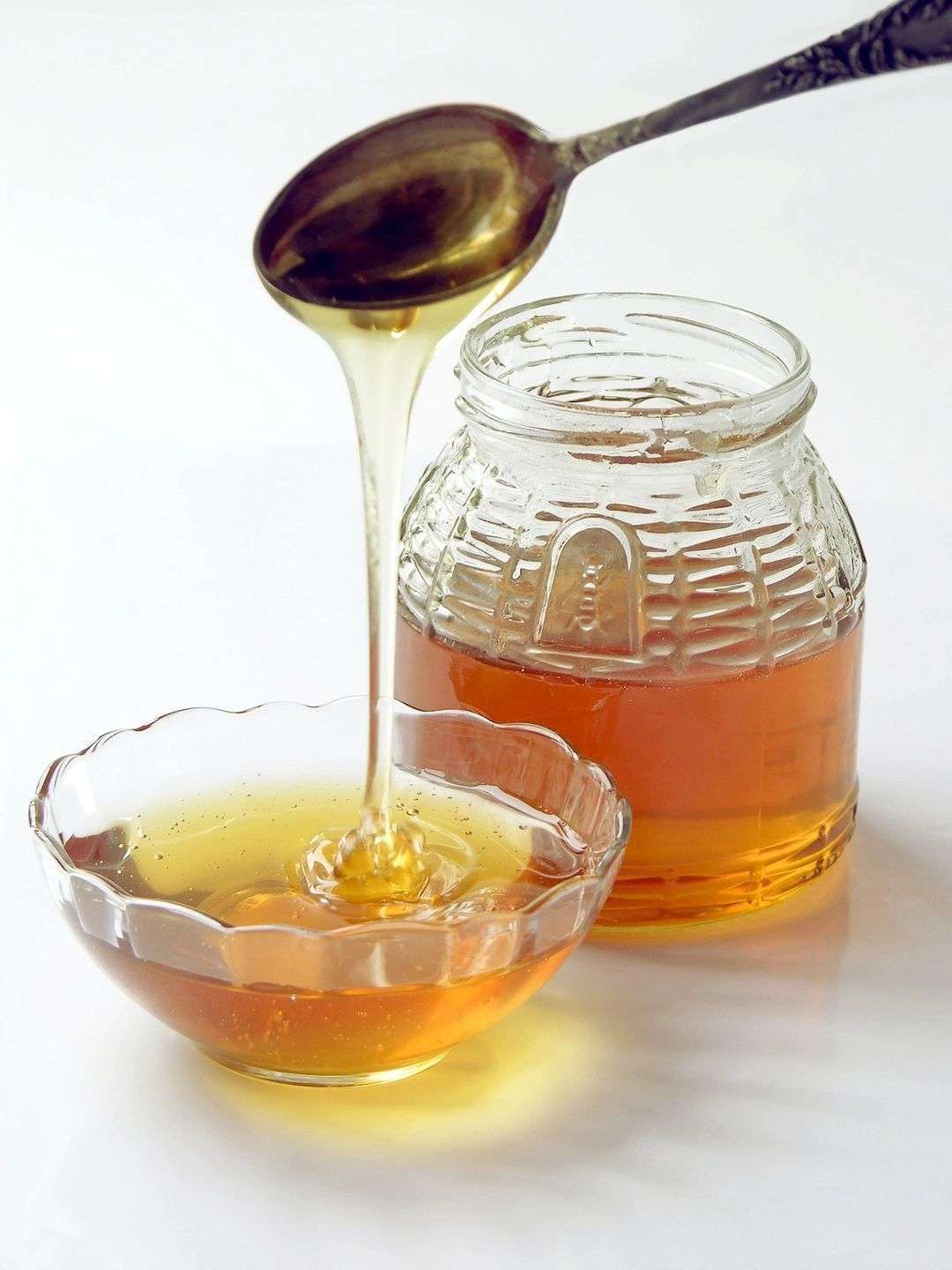raw honey
 table div table+table+table+table+table+table+table+table+table+table+table+table+table+table+table+table+table+table+table+table+table+table+table+table+table+table+table+table+table+table+table+table+table+table+table div table{width:100%;padding:0}table div table+table+table+table+table+table+table+table+table+table+table+table+table+table+table+table+table+table+table+table+table+table+table+table+table+table+table+table+table+table+table+table+table+table+table div table img{width:96.23%;padding:0;float:none}table div table+table+table+table+table+table+table+table+table+table+table+table+table+table+table+table+table+table+table+table+table+table+table+table+table+table+table+table+table+table+table+table+table+table+table div table td{width:100%;padding:0 1.88% 18px}/* styles *//* styles */ Kind regards, Your Team at Alegre Farm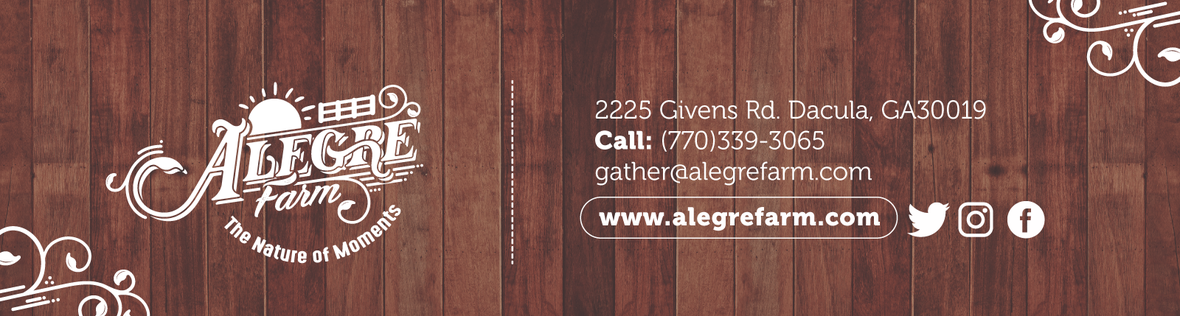Like   Tweet   Pin   +1   in